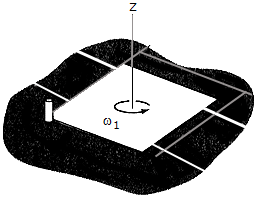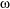# Engineering Mechanics - PKRB: Impulse and Momentum - Discussion

### Discussion :: PKRB: Impulse and Momentum - General Questions (Q.No.7)

7.The square plate, where a = 0.75 ft, has a weight of 4 lb and is rotating on the smooth surface with a constant angular velocity of1 = 10 rad/s. Determine the new angular velocity of the plate just after its corner strikes the peg P and the plate to rotate about P without rebounding.

 [A].2 = 6.67 rad/s [B].2 = 2.50 rad/s [C].2 = 10.00 rad/s [D].2 = 5.00 rad/s

Explanation:

No answer description available for this question.